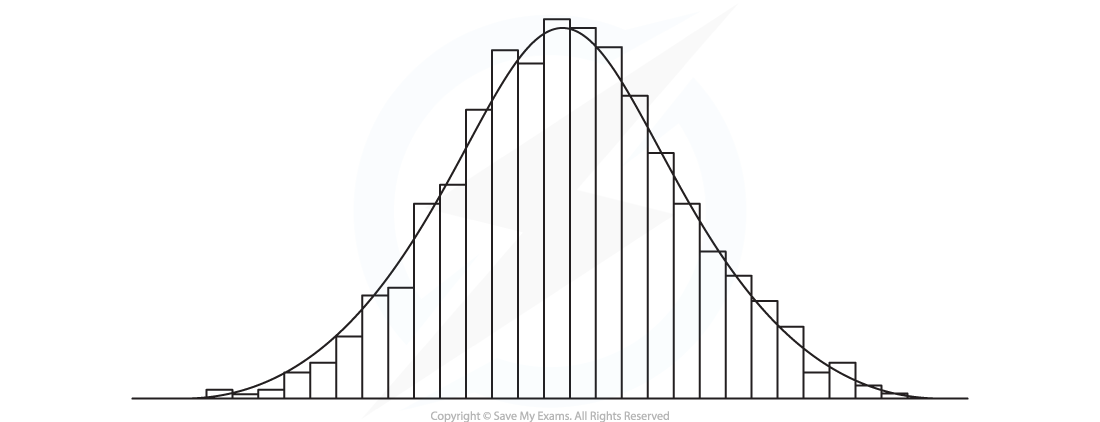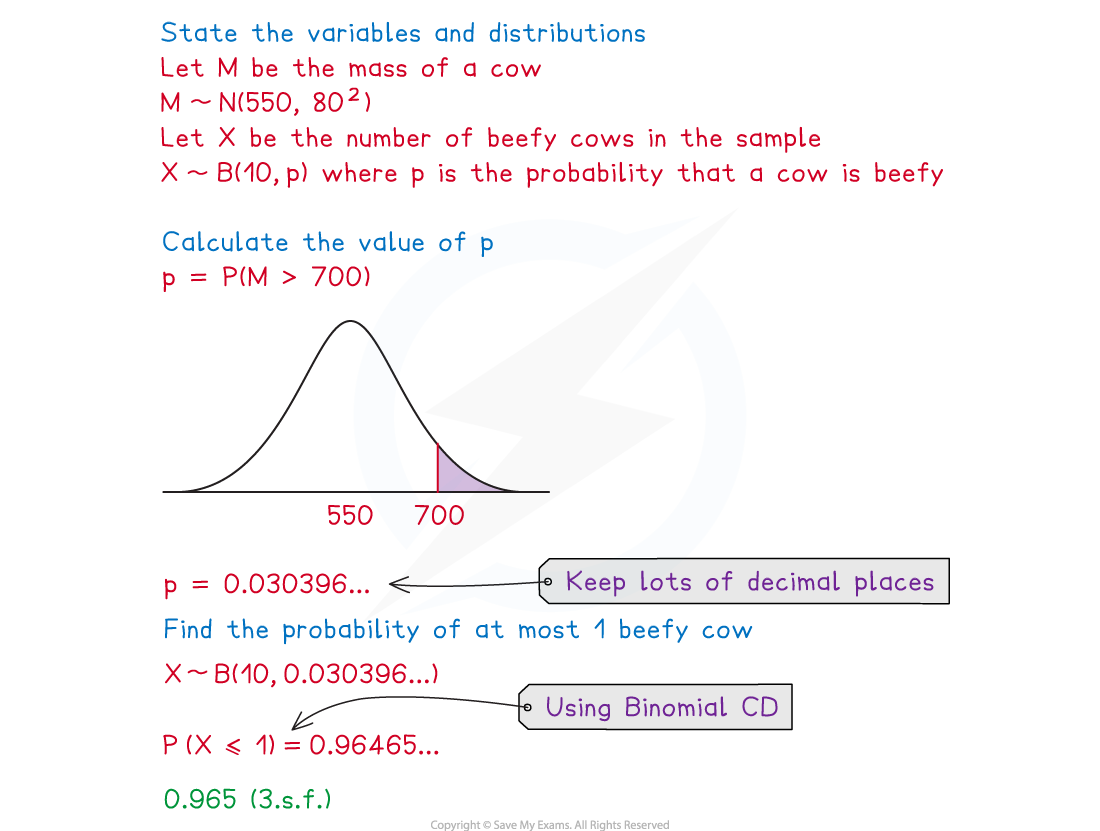# AQA A Level Maths: Statistics复习笔记4.4.1 Modelling with Distributions

### Modelling with Distributions

#### When should I use a binomial distribution?

• A random variable that follows a binomial distribution is a discrete random variable
• A binomial distribution is used when the random variable counts something
• The number of successful trials
• The number of members of a sample that satisfy a criterion (satisfying the criteria can be seen as a successful trial)
• There are four conditions that X must fulfil to follow a binomial distribution
• There is a fixed finite number of trials (n)
• The trials are independent
• There are exactly two outcomes of each trial (success or failure)
• The probability of success (p)  is constant

#### When should I use a normal distribution?

• A random variable that follows a normal distribution is a continuous random variable
• A normal distribution is used when the random variable measures something and the distribution is:
• Symmetrical
• Bell-shaped
• A normal distribution can be used to model real-life data provided the histogram for this data is roughly symmetrical and bell-shaped
• If the variable is normally distributed then as more data is collected the outline of the histogram should get smoother and resemble a normal distribution curve#### Can the binomial distribution and the normal distribution be used in the same question?

• Some questions might require you to first use the normal distribution to find the probability of success and then use the binomial distribution
• These questions normally involve some sort of sampling
• The key is to make sure you are very clear about what each parameter/variable represents

#### Worked Example

In a population of cows, the masses of the cows can be modelled using a normal distribution with mean 550 kg and standard deviation 80 kg. A farmer classifies cows as beefy if they weigh more than 700 kg. The farmer takes a random sample of 10 cows and weighs them.

Find the probability that at most one cow is beefy.#### Exam Tip

• Always state what your variables and parameters represent.  Make sure you know the conditions for when each distribution is (or is not) a suitable model.